2.7 Linear Equations:

Is it not interesting to solve problems similar to?

1.      If sum of three consecutive even numbers is 252, which are they? (80,82,84 ? 70,72,74 ? . . . )

2.      If the length of a rectangle is 4cms more than breadth and the perimeter is 11cms more than breadth. What is the length and breadth of the rectangle?

3.      A traveler on a pilgrimage  spends half the amount in Prayaga, 2/9 th of the remaining amount in Kashi, 1/4 th of the remaining amount towards taxes, 6/10 th of the remaining amount in Gaya. If he brings back 63 Nishkas ( A unit of measurement of money), tell me the total amount taken  by him( Lilavati shloka 55)

Definition : Equation is statement of equality of two algebraic expressions involving one or more unknown quantities (called Variables).

The expression on Left Hand Side is called (LHS) and expression on Right Hand Side is called RHS.

Observations:

Is it not true that 6=6  ?  ======è  (1)

Here Left hand side (LHS) is 6 and Right hand side is also 6 and both sides are equal

We thus say LHS=RHS (as both sides are equal)

Let us add the same number 2 to both sides of  (1)

We get LHS =6+2=8  and also RHS = 6+2 =8

We still notice that LHS= RHS (as both sides are equal)

Let us subtract the same number 3 from both sides of (1)

We get LHS= 6-3 =3 and RHS = 6-3 =3

We still notice that LHS=RHS (as both sides are equal)

Let us multiply both sides of (1) by the same number 6

We get LHS= 6*6=36 and RHS= 6*6 =36

We still notice that LHS=RHS (both sides are equal)

Let us divide both sides of  (1) by  the same number 3

We get LHS = 6/3=2 and RHS = 6/3=2

We still notice that LHS=RHS (both sides are equal)

Properties of Equality (Axioms)

1. Equality does not change when we add same quantity to both sides

2  Equality does not change when we subtract same quantity from both sides

3. Equality does not change when we multiply both sides by same quantity

4. Equality does not change when we divide both sides by same non-zero quantity

If we carry out any of the above mentioned operations on an equal statement (LHS=RHS), the equality (LHS+RHS) still holds well even after the operation.

Definition : An equation  containing only linear polynomial or variable in first degree  is called ‘linear equation’

Eaxmples  :   x+2 =5, 3*(a-5) =6, ½ x -4/5 = 3x+7   are all linear equations

x2-4 =0 is not a linear equation(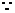Its degree is 2)

Example 1:

Let us consider the statement x-3 = 1 where x is a variable.

This statement can be explained as “find the value of x, such that, when 3 is subtracted from that number(x) we should get the result as 1”

Let us substitute the following values for x as in the statement x-3 =1

1. Can x be 1? No, because 1-3 which is -2, is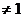2. Can x be 2 ? No, because 2-3 which is -1, is3. Can x be 5? No, because 5-3 which is 2,   is4. Can x be 4? Yes because 4-3=1.

What are we doing?

We are trying to find the value of x by trial and error method which is time consuming and is not the most effective way.

If so, then how do we find out the value of x mathematically?

Let us add 3 to both sides of the statement. Then we get x-3+3= 1+3 so we get x+0 = 4.

What did we do ?

We added the same number to both sides of the statement as per 1st  Axiom.

Why did we choose the number 3 to be added?

We wanted only x to be present on LHS and no other number along with x.

Example 2:Find the value of x such that 6x+4 = 3x+10

Here LHS =6x+4 and RHS= 3x+10

Step1:

Subtract 3x from both sides (Why? We want RHS to contain only the constant and not the variable) then we get

RHS = 3x+10-3x= 10

LHS = 6x+4-3x = 3x+4

From 2nd Axiom, RHS=LHS

Step 2:

Subtract 4 from both sides (Why? We want LHS to have only the variable part and not the constant) then we get

LHS = 3x+4-4=3x

RHS = 10-4 = 6

From 2nd Axiom, LHS=RHS

Step 3

Divide both sides by 3 (Why? We want LHS to have only variable with co-efficient=1) then we get

LHS = 3x/3 =x

RHS = 6/3 =2

From 2nd Axiom, LHS=RHS

Thus we have x=2

What did we do in first two steps?

We first subtracted 3x from both sides and then the constant (number) 4.

This is same as saying add additive inverse of 3x (i.e.  -3x) and additive inverse of 4 (i.e. -4) to both LHS and RHS

We transposed (changed)   3x from one side to another side of the equation with change in sign and then in 2nd step, transposed 4 from one side to another side of equation with change in sign

Let us summarise our steps

 Step Statement Explanation 1 6x+4= 3x+10 Given equation: 2 6x+4-3x =10 i.e. 3x+4 =10 Transposition of 3x from right  side to left side  with sign changed 3 3x= 10-4 i.e. 3x =16 Transposition of 4 from left side to  right side with  change in sign 4 x=2 Simplification(Divide both sides by 3)

Verification:

Let us replace x by 2 in the equation (1)

LHS =6*2+4 = 16 and

RHS = 3*2+10 =16

Since LHS = RHS=16,    x=2 is the correct answer.

Definition: Finding the value of variable which when substituted in the equation makes two sides (LHS and RHS) equal, is called ‘solution’ to the equation.

In the above case x =2 is the solution to the equation. You may notice that x=1 is not a solution to the above equation (because when 1 is substituted for x, we get LHS = 10 and RHS=13 and therefore LHS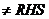)

2.7 Problem 1 : Solve (Find value of x) in

5*(2x-3) = 2*(3x-7)

Solution:

 Step Statement Explanation 1 5*(2x-3) = 2*(3x-7) Given equation: 2 10x -15 = 6x -14 Simplification 3 10x -6x= -14+15 Transposition of 6x,15 from one side to  another with sign change 4 4x = 1:i,e x = ¼ Simplification

Verification:

Substitute 1/4 for x in the given equation (1)

LHS = 5*(2*1/4 -3)  =  5*(1/2-3) = 5*(-5/2) = -25/2

RHS = 2*(3*1/4-7)  = 2*(3/4-7) = 2*(-25/4)  = -25/2

Since LHS= RHS  = -25/2,  x =1/4 is the correct answer

2.7 Problem 2 : Solve for x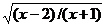= 1/2

Solution:

Get the squares of both sides

(x-2)/(x+1) = 1/4

On cross multiplication we get

4(x-2) = x+1

I.e. 4x – 8 = x+1 (simplification)

I.e. 4x –x = 1+8  ( Transposition)

I.e.  3x = 9x=3

Verification:

Substitute x=3 in the given problem to note that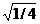= 1/2

2.7 Problem 3: Find three consecutive even numbers whose sum is 252

Solution:

Step 1 : Let x be the first  even number

Step 2 : Then the next two consecutive even numbers are x+2 and x+4

Step 3 : Sum of these three consecutive  even numbers are x+(x+2)+(x+4) = 3x+6

LHS = 3x+6

RHS = 252(Given data)

We need to solve 3x+6 = 252

On transposition we get 3x = 252-6=246 and thus x = 82

The three numbers are 82(=x), 84(=x+2) and 86(=x+4)

Verification:

82, 84, 86 are three consecutive even numbers

and their sum is = 82+84+86 which is = 252

2.7 Problem 4: A steamer goes downstream from one port to another in 9 hours. It covers the same distance upstream in 10hours. If the speed of stream be 1km per hour, find out the distance between ports

Solution:

 Step1: Let the speed of steamer in still water be x km per hour. The speed of downstream = (x+1) km per hour. The speed in upstream = (x-1) km per hour. The distance covered in 9hours while going down =9(x+1). The distance covered upstream in 10hours = 10(x-1)   Step 2: Since distance between 2 ports is same (going upstream or going downstream) 9(x+1) = 10(x-1)   Step3: We need to solve the equation 9x+9 =10x-10i.e. 9+10 =10x-9x(By transposition) Therefore 19=x .Substitute this value in 9(x+1) The distance covered while in downstream =9(x+1) = 9*(19+1) =9*20= 180km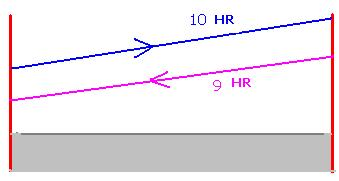Verification:

Speed of steamer while going downstream = (distance/time) – (speed of stream) = (180/9)-1   = (20-1) km/hr=19km/hr

Speed of steamer while going upstream      = (distance/time) + (speed of stream) = (180/10) +1 = (18+1) km/hr=19km/hr

Since both are same, our solution is correct.

2.7 Problem 5: A number consists of 2 digits. The digit in tens place is 2 times the digit at the units place. The number formed by reversing the digits is 27 less than the original number. Find the original number

Solution:

 1)Let x be the digit in unit place.  Since the digit in 10’s place is 2 times the digit in units place, it has to be2x. Since the number consists of 2 digits, its value = 10*digit in 10’s place + digit in units place Thus the number = 10*2x+x. =20x+x  -------------è (1) When we reverse the digits of this number we get the reverse number x 2x(x in tens place and 2x in units place) whose value= 10* digit in 10’s place + digit in units place = 10*x+2x =10x+2x                       --------------è reversed number It is given that reversed number = oldnumber-2710x+2x  = 20x+x-27 ie 12x  = 21x-27 ie 27 = 21x-12x (By transposition of 12x and 27) i,e 27 =9xx = 3. Hence the original number is 63(digit in tens place is twice the digit in units place)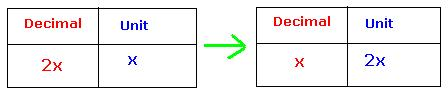Verification:

Since the original number is 63, the reversed number is 36. 36 is 27 less than 63.

Thus reversed number is 27 less than original number (which is as given in the problem).

Hence we have solved the problem correctly

2.7 Problem 6:  The length of a rectangle is 4cms more than breadth and the perimeter is 11cms more than breadth. Find the length and breadth of the rectangle.

Solution: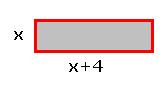Step 1: let x be the breadth. Then length = x+4.

We know perimeter P = 2*length + 2*breadth = 2(x+4)+2x   =2x+8+2x          --------------èEquation  (1)

We are also given that perimeter is 11cm more than breadth ( ie P =  x+11)    --------------è Equation (2)

Step 2 : Both are perimeters of the same rectangle and hence they have to be equal:

So Equation (1) = Equation (2)

I.e. 2x+8+2x = x+11:

I.e.4x+8 = x+11

I.e. 4x-x = 11-8(By transposition of x and 8):

I.e.3x = 3: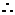x = 1.

Therefore breadth =1cm and length=5cms(x+4)

Verification:

The perimeter of rectangle = 2*length + 2*breadth = 2*5+2*1 = 10+2 = 12cms = 11cms +1cm = 11cms +breadth.

Hence we have solved the problem correctly.

2.7 Problem 7:  In a fraction, twice the numerator is 2 more than the denominator. If 3 is added to each numerator and denominator, the new fraction is 2/3. Find the original fraction

Solution:

Step1: Let x be the numerator

It is given that twice the numerator is two more than the denominator ( That is  to say 2*numerator  = denominator+2)

Hence denominator =2x-2.

Thus the original fraction is x/2x-2

When 3 is added to denominator the new denominator= (2x-2) +3=2x+1

When 3 is added to numerator the new numerator = x+3

Thus the new fraction is (new numerator)/ (new denominator) = (x+3)/ (2x+1)

Since the new fraction is 2/3 we have

Step2 : 2/3 = (x+3)/(2x+1)  --------------è(1)

By Multiplying both sides of (1) by (2x+1), we get

2*(2x+1)/3 = x+3               --------------è(2)

By multiplying both sides of (2) by 3, we get

2*(2x+1) =3(x+3)

I.e. 4x+2 =3x+9 and on transposition of 3x and 2 we get

4x-3x= 9-2

I.e. x= 7The original denominator = 2x-2 =14-2=12

Thus the original fraction was 7/12

Verification:

To get the new fraction, add 3 to both numerator and denominator so we get the new fraction as 10/15 which is 2/3

This shows that we solved the problem correctly

2.7 Problem 8:  Divide 32 in to two parts such that if the larger is divided by the smaller, the quotient is 2 and the reminder is 5

Solution:

 Let x be the larger number and hence 32-x will be the smaller number We know dividend = quotient*divisor + reminder Therfore we need to solve the equation: x/(32-x) = 2+ 5(as reminder)    Exercise : Solve   x = 2(32-x) +5 to get the answer x=23 and other number as 9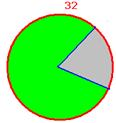2.7 Problem 9:  Find a positive value of variable x for which the given equation   x2-9/( x2+5) =  -5/9 is satisfied

Solution:

Given equation is x2-9/( x2+5) =  -5/9

On cross multiplication we get 9(x2-9) = -5(x2+5)

On simplification we get 9x2-81 = -5x2 -25

On transposition 14x2 = 56x2 = 4x =  +2 or -2

Verification:

Substitute x=2 in the given equation to arrive at LHS = -5/9 which is RHS and hence x=2 is correct

2.7 Problem 10:  Of the group of butter flies, 1/5 th proceeded towards Kadamba tree, 1/3 of the group  proceeded towards Shilindhra tree,  thrice the differences of the above two groups proceeded towards Kutaja tree. If the remaining only one was flying around attracted by aroma of ketakamalati flowers, Lilavati tell me the count of butter flies.

( Lilavati Shloka 56)

Solution:

Let  the  number of butter flies be x.

 Step To where How many 1 Kadamba tree (x/5) 2 Shilindhra tree (x/3) 3 differences of the above (x/3) – (x/5) = (2x/15) 4 Kutaja tree 3*(2x/15)=(2x/15) 5 Remaining 1x- {(x/5)+(x/3)+(2x/5) =1{15x-(3x+5x+6x)/15} =1x=15

Verification:

15=3+5+6+1

2.7 Problem 11:  A traveler  on pilgrimage  spends half the amount in Prayaga, 2/9 th of the remaining amount in Kashi, 1/4 th of the remaining amount towards taxes, 6/10 th of the remaining amount in Gaya. If he brings back 63 Nishkas (A unit of measurement of money), tell me the total amount taken  by him( Lilavati shloka 55)

Solution:

Let the  number of butter flies be x.

 Step To where Towards How much Balance 1 Prayaga (x/2) x-(x/2) = (x/2) 2 Kashi (2/9)*(x/2)=(x/9) (x/2)-(x/9) = (7x/18) 3 Taxes (1/4)*(7x/18) =(7x/72) (7x/18) - (7x/72)= (21x/72) =(7x/24) 4 Gaya (6/10)*(7x/24)=(7x/40) (7x/24)- (7x/40) ={(35x-21x)/120}=(7x/60) 5 Remaining 63(7x/60) =63x=540

Verification:

Try yourself

2.7 Summary of learning

 No Points studied 1 Transposition of terms from LHS to RHS with  change in sign 2 Simplify LHS and RHS such that variables are on one side and constants are on the other side.